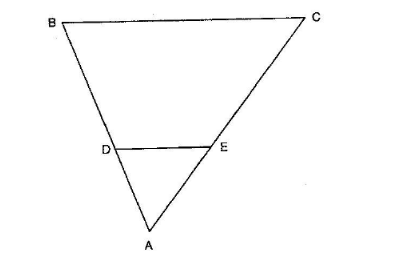# In the given figure, DE || BC in ∆ABC such that BC = 8 cm, AB = 6 cm and DA = 1.5 cm. Find DE.

Question:

In the given figure, DE || BC in ∆ABC such that BC = 8 cm, AB = 6 cm and DA = 1.5 cm. Find DE.Solution:

Given: In ∆ABC, DE || BC. BC = 8 cm, AB = 6 cm and DA = 1.5 cm.

To find: DE

So,

$\frac{\mathrm{BC}}{\mathrm{DE}}=\frac{\mathrm{AB}}{\mathrm{DA}}$

$\frac{8}{D E}=\frac{6}{1.5}$

$\mathrm{DE}=\frac{8 \times 1.5}{6}$

$\mathrm{DE}=2 \mathrm{~cm}$